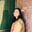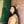Trusted answers to developer questions
Trusted Answers to Developer Questions

Related Tags

c

# What is imaxdiv() in C?Umme Ammara

Grokking Modern System Design Interview for Engineers & Managers

Ace your System Design Interview and take your career to the next level. Learn to handle the design of applications like Netflix, Quora, Facebook, Uber, and many more in a 45-min interview. Learn the RESHADED framework for architecting web-scale applications by determining requirements, constraints, and assumptions before diving into a step-by-step design process.

The imaxdiv function is a C library function that performs integer division on values of the intmax_t type.

The intmax_t is the longest integer type in C. It is the same as the long or the long long type.

The following illustration shows an example of integer division:

To use the imaxdiv function, the inttypes.h header file needs to be included in the program, as shown below:

#include <inttypes.h>


## Syntax

The imaxdiv function is declared as follows:

## Parameters

The imaxdiv function takes two arguments (dividend and divisor) of the intmax_t type.

## Return Value

The imaxdiv function returns a structure of the imaxdiv_t type. It is an object that stores the quotient and the remainder, both of the intmax_t type.

The imaxdiv_t object contains:

• intmax_t quot

• intmax_t rem

If the divisor is zero, the compiler raises the divide by zero exception.

## Example

The code below shows the use of the imaxdiv function in C:

Note that the imaxdiv function is equivalent to the div function in C. However, the div function takes the int type as arguments and returns the div_t type, whereas the imaxdiv function takes the intmax_t type as arguments and returns the imaxdiv_t type.

#include <stdio.h>#include <inttypes.h>int main() {  //Declare dividend and the divisor   intmax_t dividend = 1234567;  intmax_t divisor = 4560;    //Call the imaxdiv function  imaxdiv_t result;  result = imaxdiv (dividend, divisor);  //Access the remainder and quotient  intmax_t remainder = result.rem;  intmax_t quotient = result.quot;  //Display results  printf ("The Quotient is: %ji\n", remainder);  printf ("The Remainder is: %ji\n", quotient);    return 0;}

RELATED TAGS

c

CONTRIBUTORUmme Ammara